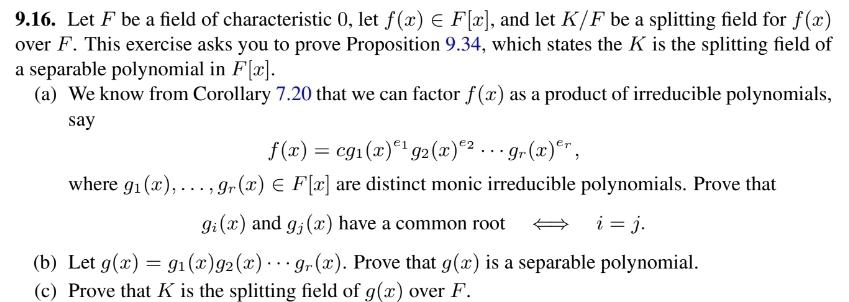# (Solved): 9.16. Let F be a field of characteristic 0 , let f(x)F[x], and let K/F be a splitting field for ...9.16. Let be a field of characteristic 0 , let , and let be a splitting field for over . This exercise asks you to prove Proposition 9.34 , which states the is the splitting field of a separable polynomial in (a) We know from Corollary 7.20 that we can factor as a product of irreducible polynomials, say where are distinct monic irreducible polynomials. Prove that and have a common root . (b) Let . Prove that is a separable polynomial. (c) Prove that is the splitting field of over .

We have an Answer from Expert